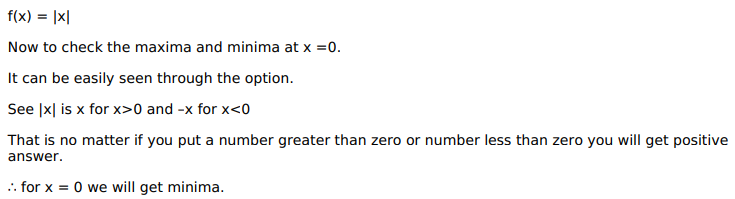# Solve this following

Question:

Mark $(\sqrt{)}$ against the correct answer in the following:

$f(\mathrm{x})=|\mathrm{x}|$ has

A. minimum at $x=0$

B. maximum $x=0$

C. neither a maximum nor a minimum at $x=0$

D. none $f$ these

Solution: